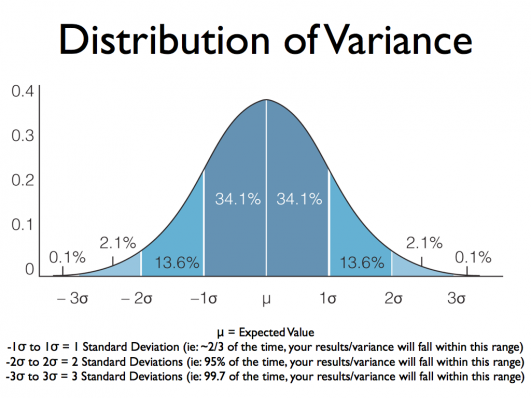# Scores on a certain IQ test are approximately normally distributed with a mean of 120 and a standard deviation of 15. In what range do 68% of all scores lie?

Dear Zachary Chasse,

A visual may help you out here...because in a normal distribution of data, the points will cluster in a classic bell-shaped curve around one average value - known as the mean, and symbolized as lower-case mu (Greek letter).

And one standard deviation from that mean, designated lower-case sigma, will contain 68.2% of all the values. So if the standard deviation is large, the bell will be short and spread out; if small, then the curve will be tall and narrow.

As you see, your standard deviation of fifteen will thus contain IQ scores ranging between 105 and 135.

http://www.wikihow.com/Calculate-Standard-Deviation3 People thanked the writer.